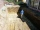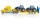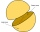# Algebra - math word problems

#### Number of problems found: 2801

• Work togetherTwo bricklayers plastering a wall. The first would plaster it in 8 hours, the second in 12 hours. How many hours will they be done with the work if they work together?
• The tentCalculate how much cover (without a floor) is used to make a tent that has the shape of a regular square pyramid. The edge of the base is 3 m long and the height of the tent is 2 m.
• Slow saving in banksHow long will it take to save € 9,000 by depositing € 200 at the beginning of each year at 2% interest?
• What isWhat is the annual percentage increase in the city when the population has tripled in 20 years?
• Age of familyThe age of father and son is in the ratio 10: 3. The age of the father and daughter is in ratio 5: 2. How old are a father and a son if the daughter is 20?
• Magnified cubeIf the lengths of the edges of the cube are extended by 5 cm, its volume will increase by 485 cm3. Determine the surface of both the original and the magnified cube.
• DriversDriver Franta and driver Karel are friends. They leave the start-stop both at the same time in the afternoon - at 13.30. When will they meet again if Franta goes around his route in 50 minutes and Karel in 40 minutes?
• DitchThe excavator dug 40% of the excavation in 2 hours 20 minutes. How long does it take him to dig the whole ditch?
• Viewing angleThe observer sees a straight fence 60 m long at a viewing angle of 30°. It is 102 m away from one end of the enclosure. How far is the observer from the other end of the enclosure?Calculate the surface of a quadrilateral pyramid, which has a rectangular base with dimensions a = 8 cm, b = 6 cm and height H = 10 cm.
• Side lengthsIn the triangle ABC, the height to the side a is 6cm. The height to side b is equal to 9 cm. Side "a" is 4 cm longer than side "b". Calculate the side lengths a, b.
• The number 72The number 72 increase by 25%. By how much % will you have to reduce the number you created to get the number 72 again?Adam has half the money in his right pocket than in his left pocket. If he transferred 40 crowns from the left pocket to the right, he would have the same in both pockets. Calculate how many crowns does Adam have in his left pocket more than in his right?
• Observation towerFrom the observation tower at a height of 105 m above sea level, the ship is aimed at a depth angle of 1° 49´. How far is the ship from the base of the tower?
• Pentagonal prismThe regular pentagonal prism is 10 cm high. The radius of the circle of the described base is 8 cm. Calculate the volume and surface area of the prism.
• Five tractorsFive tractors plow the fields in 4.8 hours. How long will it take to plow the field when, after an hour and a half of work, two tractor drivers have been called up for another task?
• Triangle from medianCalculate the perimeter, content, and magnitudes of the remaining angles of triangle ABC, given: a = 8.4; β = 105° 35 '; and median ta = 12.5.
• Two hemispheresIn a wooden hemisphere with a radius r = 1, a hemispherical depression with a radius r/2 was created so that the bases of both hemispheres lie in the same plane. What is the surface of the created body (including the surface of the depression)?
• DiggersThe excavation was carried out in 4 days and 5 workers worked on it for 7 hours a day. Determine how long it would take to excavate if 7 workers worked on it 8 hours a day.
• Wire fenceThe wire fence around the garden is 160 m long. One side of the garden is three times longer than the other. How many meters do the individual sides of the garden measure?

Do you have an interesting mathematical word problem that you can't solve it? Submit a math problem, and we can try to solve it.

We will send a solution to your e-mail address. Solved examples are also published here. Please enter the e-mail correctly and check whether you don't have a full mailbox.

Please do not submit problems from current active competitions such as Mathematical Olympiad, correspondence seminars etc...